Next: d'Alembert's Paradox Up: Incompressible Inviscid Flow Previous: Bernoulli's Theorem

# Euler Momentum Theorem

Consider the stream filament shown in Figure 4.1. Letand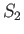be the cross-sectional areas at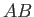and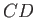, respectively, and let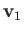and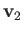be the corresponding flow velocities. Assuming the the flow is both steady and incompressible, Euler's momentum theorem, which is named after Leonhard Euler (1707-1783), states that, neglecting external forces, the resultant force due to the pressure of the surrounding fluid on the walls and ends of the filament is equivalent to forces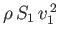and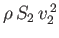acting normally outward at the endsand, respectively.

The proof is straightforward. According to Newton's second law of motion, the resultant force must produce the change in the momentum of the fluid that occupies the portion of the filament betweenandat any given instant of time,. Suppose that at time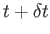the fluid in question occupies the portion of the filament between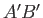and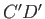. The momentum of the fluid in question has then increased by the momentum of the fluid betweenand, and decreased by the momentum of the fluid betweenand. Hence, there has been a gain of momentum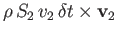at, and a loss of momentum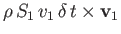at. Thus, the net rate of charge of momentum consists of a gain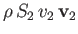at, and a loss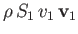at. This net rate of change is produced solely by the thrusts acting on the walls and ends of the filaments. It follows that these thrusts are equivalent to the forcesandacting normally outward atand, respectively.

If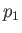andare the pressures atand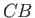, respectively, then the thrusts acting normally inward on the ends of the filament are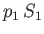atand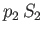at. According to Euler's theorem, the thrusts exerted on the walls plus the thrusts acting on the ends are equivalent to the normal outward forcesatandat. It follows that the thrusts exerted by the walls on the fluid are equivalent to the normal outward forces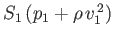atand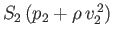at. Conversely, the thrusts exerted by the fluid on the walls are equivalent to normal inward forcesatandat.

Note, finally, that the Euler momentum theorem obviously also applies to a stream tube, as long as the flow through the ends of the tube is uniform across the cross-section.Next: d'Alembert's Paradox Up: Incompressible Inviscid Flow Previous: Bernoulli's Theorem
Richard Fitzpatrick 2016-03-31